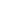• μ denotes the mean of the population, also referred to as the process mean or the process level.
 • μ0 denotes the target mean (or goal) for the population. Sometimes, the symbol X0 is used for μ0. See the American Society for Quality Statistics Division (2004). You can provide μ0 as the Target in the Known Statistics for CUSUM Chart area on the launch window.
 • σ denotes the population standard deviation.denotes an estimate of σ.
 • σ0 denotes a known standard deviation. You can provide σo as the Sigma in the Known Statistics for CUSUM Chart area on the launch window.
 • n denotes the nominal sample size for the CUSUM chart.
 • δ denotes the shift in μ to be detected, expressed as a multiple of the standard deviation. You can provide δ as the Delta in the Known Statistics for CUSUM Chart area on the launch window.
 • Δ denotes the shift in μ to be detected, expressed in data units. If the sample size n is constant across subgroups, then the following computation applies:
You can provide Δ as the Shift in the Known Statistics for CUSUM Chart area on the launch window.

Help created on 9/19/2017